# Fabian Ruehle - NewsFabian Ruehle Assistant Professor Physics and Mathematics Affiliated with IAIFI Northeastern University Dana Research Center Office 223 Boston, MA 02115 Phone: +1 617-373-8109
 Flops for Complete Intersection Calabi-Yau Threefolds [arxiv:2112.12106]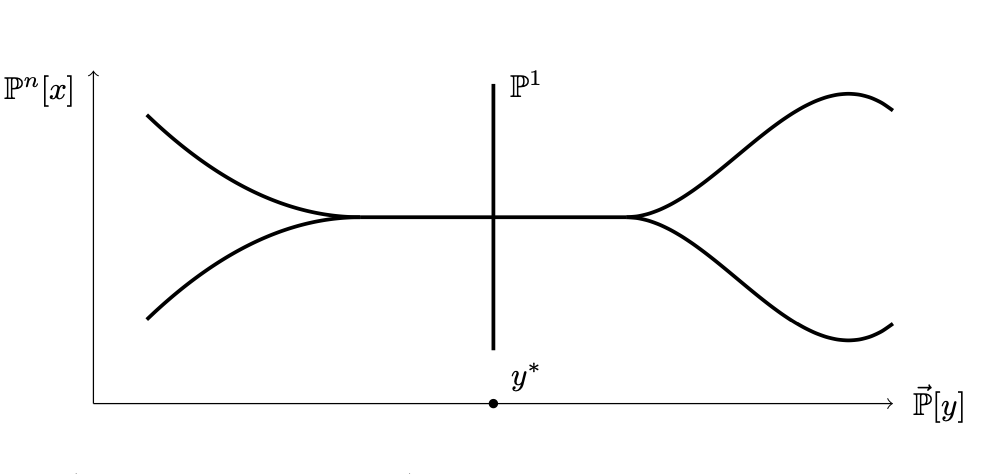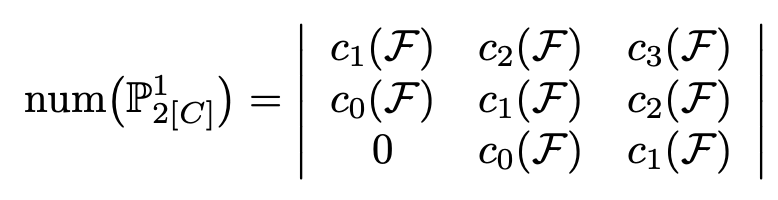We discuss flops in projective Complete Intersection Calabi-Yau manifolds. We explain that there are two different types of flops, whose presence and type can be read off from the GLSM charges. Of course, this can also be used to engineer manifolds that exhibit a certain flop type. Case 1: A GLSM U(1) charge contains only 0's and 1's. This signals the presence of flops to non-isomorphic manifolds. Case 2: A GLSM U(1) charge contains a single 2, followed by 0's and 1's. This signals the presence of flops to isomorphic manifolds. We explain in both cases how to describe the manifold as well as the singular manifold that sits in between two flops. These require moving away from projective CICYs to more general toric models, determinantal varieties (for case 1), or more complicated manifolds (in the second case). As shown in the figure, these are small resolutions of branched double covers of the "base". We also explain how to describe the flopped divisors (and in fact the isomorphism of the Picard groups) under both types of flops. We also explain how to get the number of contracted curves in the flop from either comparing the Euler characteristic or by using the Giambelli-Thom-Porteous formula (see bottom figure for an example).

more...

 Recent Developments in Line Bundle Cohomology and Applications to String Phenomenology [arxiv:2112.12107]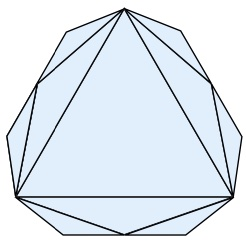This is a conference proceeding to the Nankai Symposium on Mathematical Dialogues: In celebration of S.S.Chern's 110th anniversary. We review progress in deriving closed-form expressions for line bundle cohomologies and discuss applications to string phenomenology. We also review results from the papers [2104.03325], [2108.10323], and [2112.12106] on the structure and properties of the extended Kahler cone of CICY manifolds. The figure illustrates the structure of the (convex) extended Kahler cone in the case of infinitely many flops. The extended Kahler cone actually has infinitely many subcones, which become, however, smaller and smaller (just in the original coordinate system; their diameter, as measured with the moduli space metric, actually stays the same), such that they cannot be seen in the image.

more...

 Learning Size and Shape of Calabi-Yau Spaces [arxiv:2111.01436]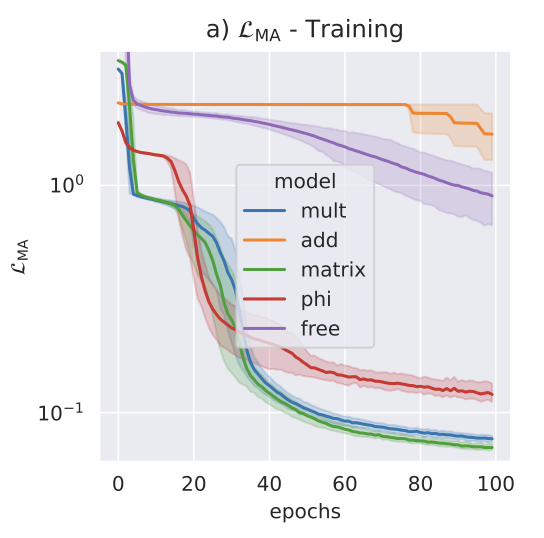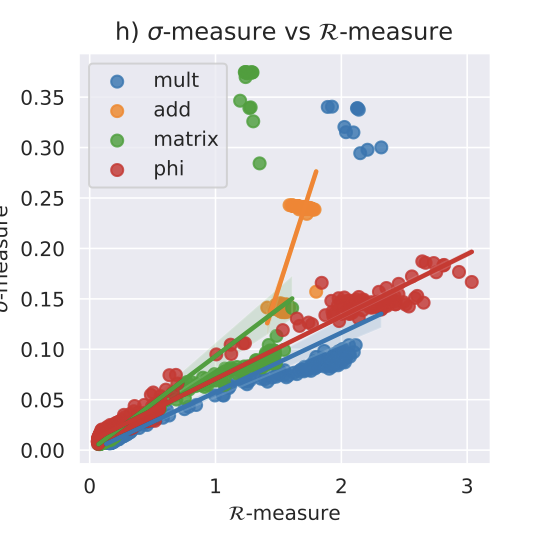For the NeurIPS ML and the Physical Sciences workshop, we presented our library cymetric to compute Calabi-Yau metrics for any projective complete intersection Calabi-Yau or toric Kreuzer-Skarke Calabi-Yau. We vastly extend to scope by implementing general point sampling methods on the CY with known distributions for any toric or projective ambient space (i.e. for any $$h^{1,1}$$). We also introduce a new architecture, the "Phi Model", which allows to find the Ricci flat metric in a fixed Kahler class. The code is written in TensorFlow, but we also provide an API for SageMath and for Mathematica. The latter allows to train a NN to approximate the CY metric with just a few lines of Mathematica code and does not require any knowledge of Python. We demonstrate the code for a few example Calabi-Yau manifolds (see top figure for how the Monge-Ampere equation gets solved to higher and higher accuracy during training for various CY metric ansatze). The code can compute many quantities related to CY metrics, including the Ricci tensor, the Ricci scalar, and the Kahler potential (in the calse of the Phi Model). The change in the Ricci scalar as a function of the change in the Monge-Ampere equation is plotted in the bottom figure. We see that once the Monge-Ampere equation is solved exactly, the metric is Ricci-flat; this had to happen, since the Calabi-Yau metric is unique, and it is very nice to see this reproduced. Interestingly, the origin is approached along a line.

more...

 Geodesics in the extended Kähler cone of Calabi-Yau threefolds [arxiv:2108.10323]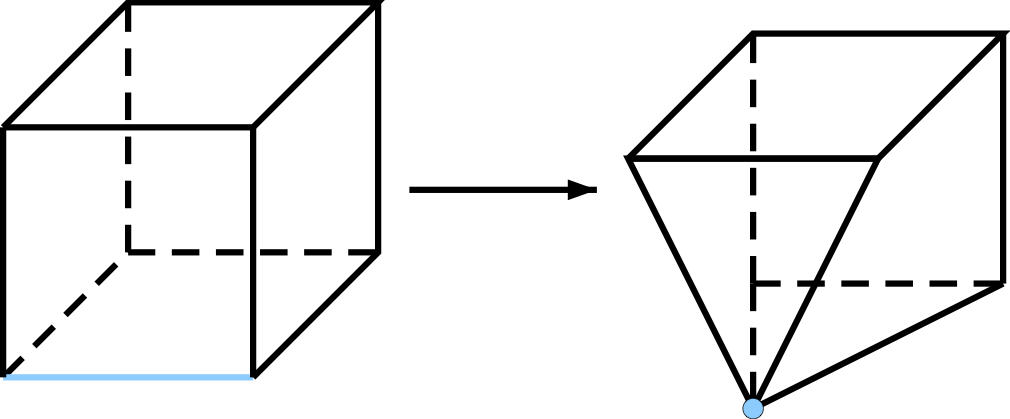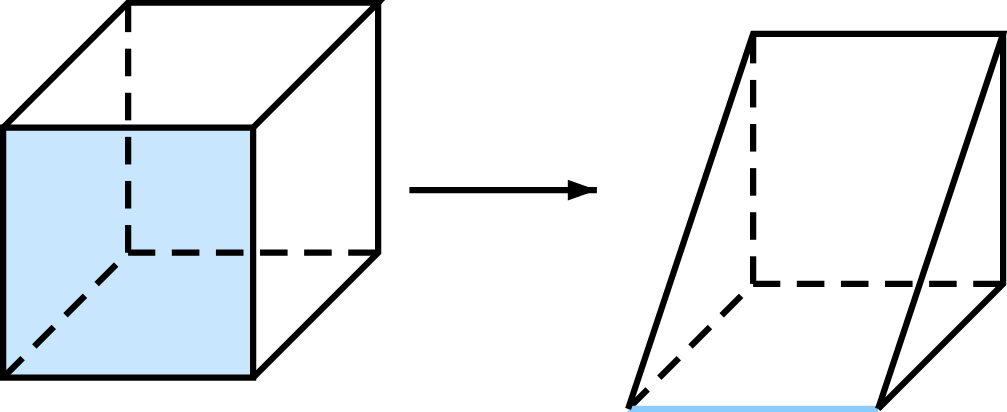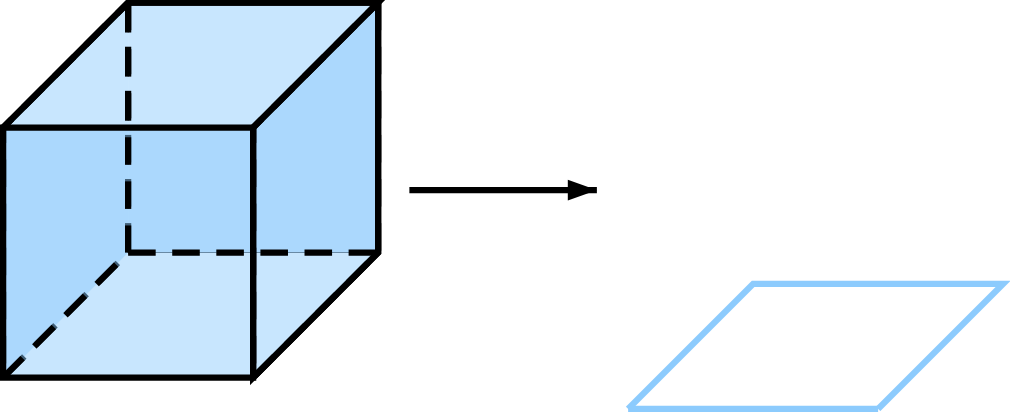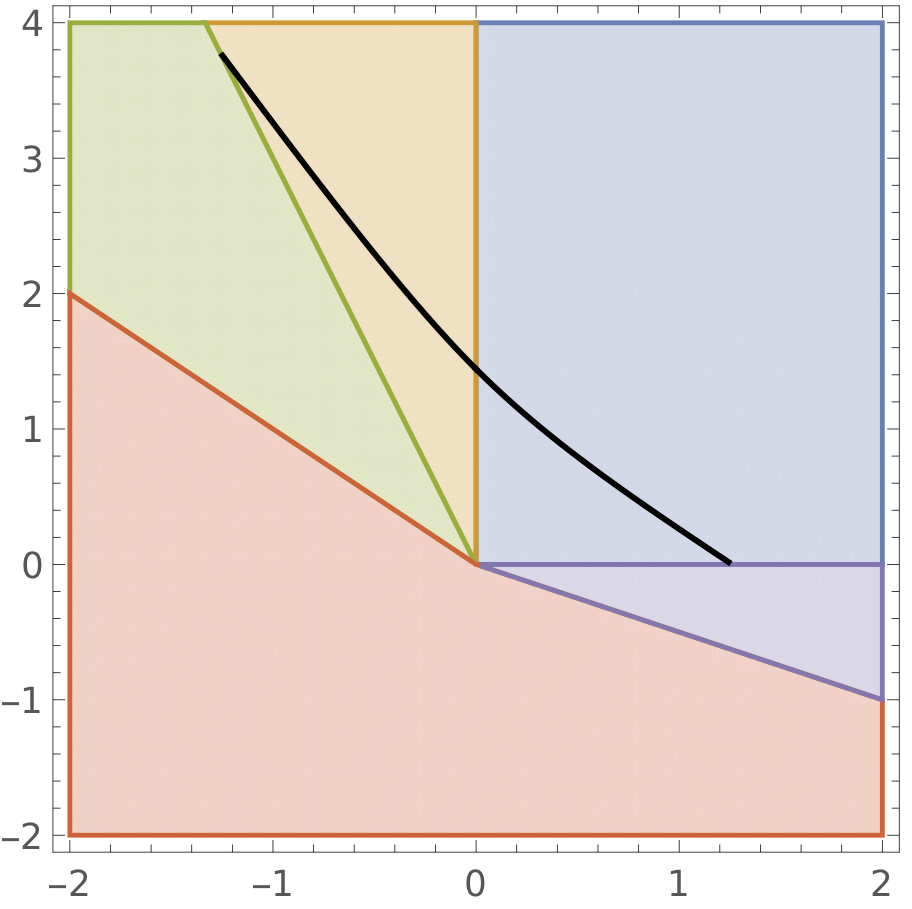We continue our study of the moduli space of a Calabi-Yau (CY) and the topology changes that can occur. We find that as one traverses the moduli space, three things can happen: Case 1: A curve collapses but the volume of divisors and the CY stays finite (see first picture). This indicates a "flop wall". One can go through this singularity and emerge on the other side in another geometry where the collapsed curve is replaced by another curve. Case 2: A divisor collapses, but the CY volume stays finite (see second picture). This indicates a somewhat mysterious boundary which we call a Zariski wall. The CY volume stays finite, but one nevertheless does not have a geometric description beyond this wall. Case 3: The volume of the whole CY collapses (see third picture). This indicates the end of the effective cone. One would need a GLSM or some other description to make sense of string theory beyond this wall. For CYs with 2 Kahler parameters in M-Theory, we can solve the geodesics equation of motion analytically (case 3 features every trigonometric function in the book...) in the vector moduli multiplet space of the theory, which essentially means solving the equations along curves of constant overall volume. We find: Case 1: These are always at finite geodesic distance. Case 3: These are always at infinite geodesic distance (this is obvious, since the volume of the CY shrinks in case c, but we are moving along curves of constant volume). Case 2: This is the strangest case. If we wanted to probe what’s beyond the Zariski wall and happily follow our geodesic, we find that the geodesic “bounces off” the Zariski wall and is reflected back into the geometric regime we came from (see fourth picture). And that bounce happens at finite distance. Moreover, this bounce is not benign: the closer we get to the wall, the more the geodesic motion accelerates, reaching infinite speed at the wall. So it crashes into the wall with infinite speed and is re-emitted into the opposite direction, also with infinite speed. Of course our theory is invalidated before reaching infinite speed, and it is suggested that an additional SU(2) theory appears at such walls. Presumably, if we could correctly include the SU(2) vector multiplet, the singularity would be cured, but we don’t attempt this in this paper.

more...

 String Pheno 2021 Conference HomepageIn the week of July 12th to July 16th 2021, Northeastern is hosting the 20th installment of the anual String Phenomenology conference, the largest conference in this field. Due to the pandemic, the conference takes place virtually. It covers a range of topics, including •   Swampland and quantum gravity conjectures •   Machine learning and AI techniques in string theory •   Formal and mathematical aspects of string compactifications •   String model building in particle physics and cosmology If you are interested, just register to receive Zoom links to the talks.

more...

 A blood atlas of COVID-19 defines hallmarks of disease severity and specificity [medrxiv]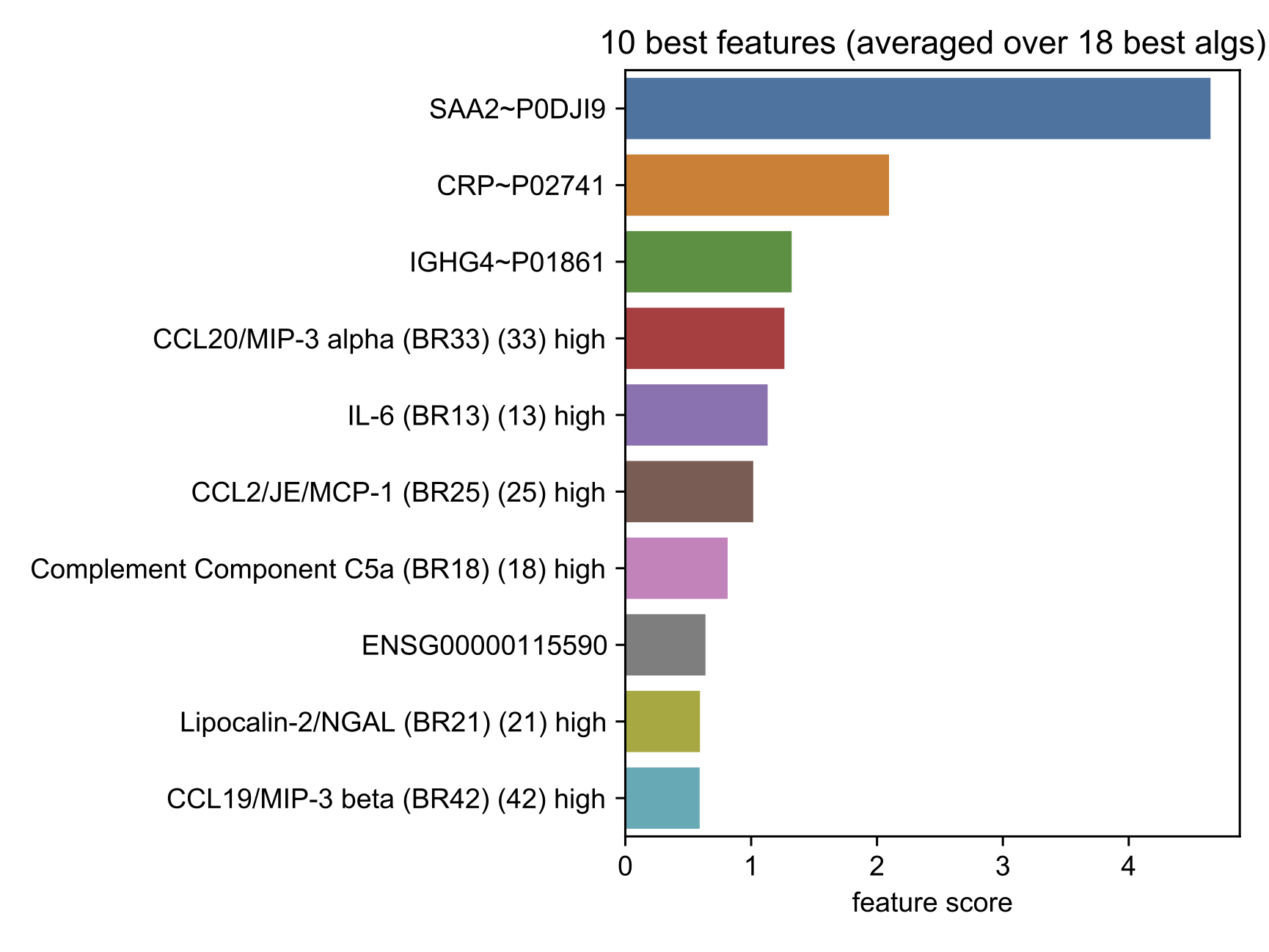In a collaboration with biologists, clinicians, and mathematicians, we studied various aspects of COVID19. I used ML to answer two questions: 1.) In collaboration with two colleagues from genomic medicine and a radiologist, and one from respiratory immunology, we studied which (combination of) clinical parameters best describes the status of hospitalized covid patients (mild, severe, critical). The analysis was carried out using unsupervised ML tools (k-means consensus clustering). The classification of the health status assigned by medical doctors matched nicely onto the clinical variables, cf. top figure, which shows three clusters for mild, severe, critical identified by the unsupervised ML algorithm. This gave us confidence that we are using the right measure to classify patients. 2.) Given the above classification in terms of clinical variables, I used different laboratory data (gained from RNA sequencing, Luminex analysis, Mass Spectrometry, ...) to to identify proteins or genes that lead to severe desease progression in covid patients. For this I first computed principal components and scored them (using feature permutation scoring) based on 20 ML classification algorithms. I then took the best-performing Prinicpal Components and reran the analysis for the proteins/genes that loaded these Components the strongest. A summary of the genes/proteins that were most important in distinguishing mild, severe, and critical patients are given in the bootom figure. Overall, I think this is a great example that demonstrates the power for transfer of knowledge across the field based on the common language of ML.

more...

 Swampland Conjectures and Infinite Flop Chains [arxiv:2104.03325]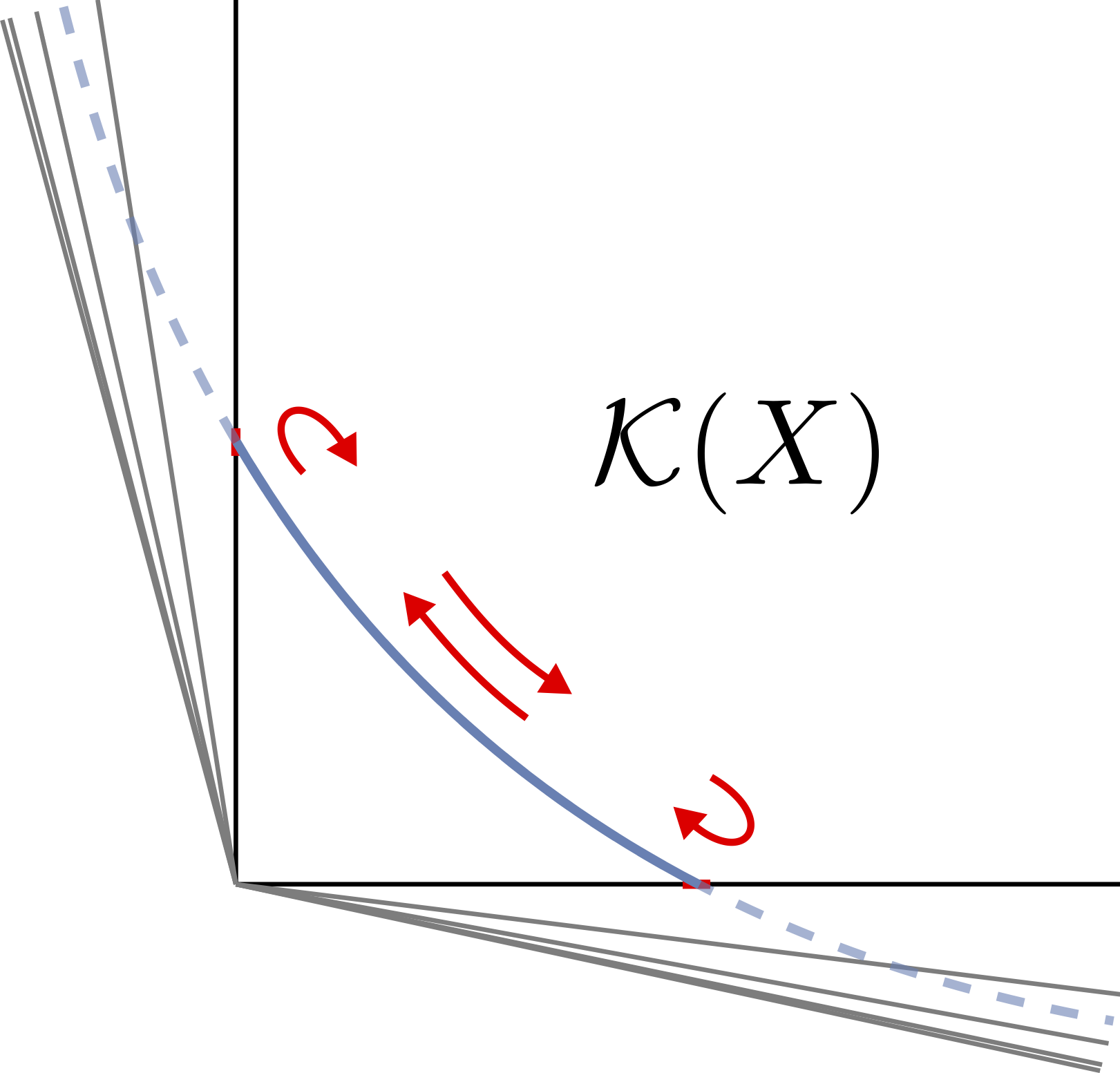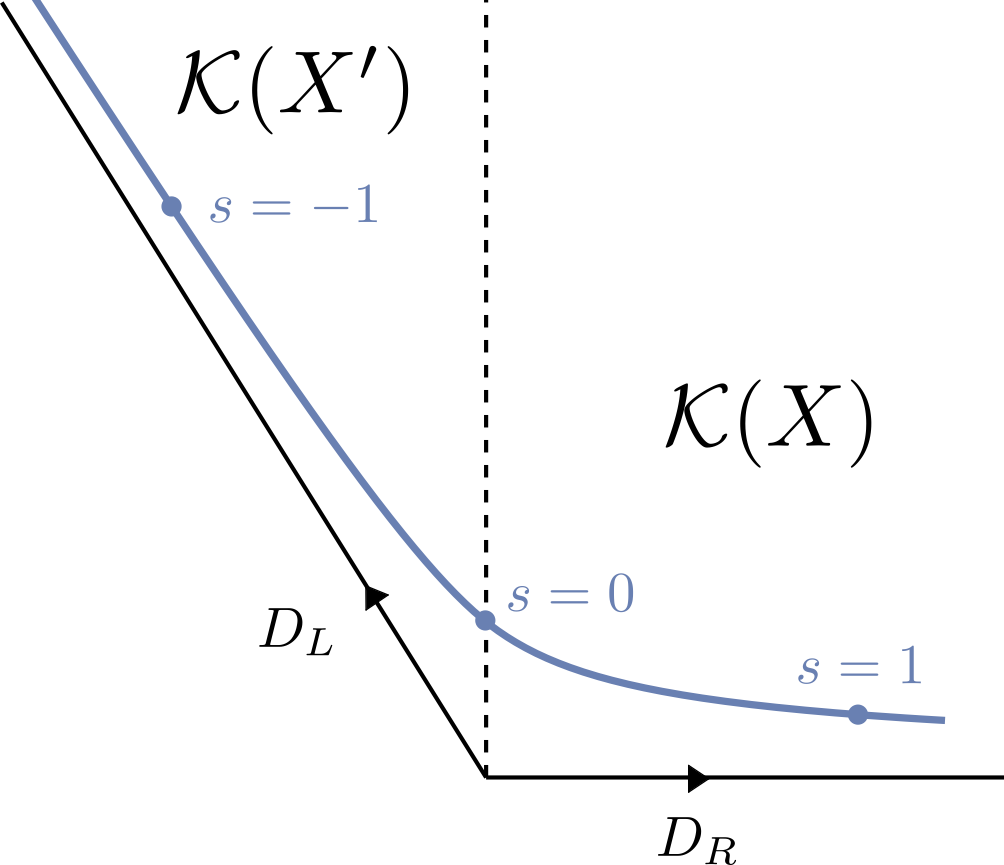As you walk through the moduli space of a Calabi-Yau (CY), the CY can change its topology. Very common topological transitions are flops. Flops can lead to new or to equivalent CYs, and there are even cases where infinitely many flops occur. This begs the question: What happens to the Swampland Distance Conjecture (SDC), i.e. which tower of states becomes light? Clearly, for flops to equivalent CYs, the entire spectrum is identical, yet the geodesic distance between equivalent points in different cones is non-zero. In fact, it can be arbitrarily large in the infinite flop case, see top figure (while the figure looks like the cones get smaller and smaller, the moduli space metric behaves such that the distance between the walls of each cone is the same; indeed, we can identify the symmetry that maps all cones onto one another). We argue that in such cases there is a remnant discrete gauged symmetry, inherited from the 11D Poincare symmetry of M-theory, which identifies all Kähler cones. Consequently, the shortest geodesic connecting two equivalent points corresponds to not moving at all. So what about the case with inequivalent flops? If there are finitely many, there is a divisor at the boundary of the Kähler cone (denoted by $$D_L$$ and $$D_R$$ in the bottom figure). Approaching a wall then means that the winding modes on this divisor become light, providing the tower of light states predicted by the SDC. This resolution is, however, not available if there exist infinitely many flops to inequivalent CYs. In these cases, there is no divisor at the "end" of the Kähler cone, and hence no associated tower can become light. In contrast to infinitely many equivalent CYs, we haven't seen such cases yet, and indeed a conjecture by Kawamata and Morrison says that they cannot occur. Conversely, the SDC applied to infinitely many inequivalent flops implies the Kawamata-Morrison conjecture. I think it's fun to use physics in this way to explore properties of CYs!

more...

 Moduli-dependent KK towers and the Swampland Distance Conjecture on the Quintic [arxiv:2103.07472]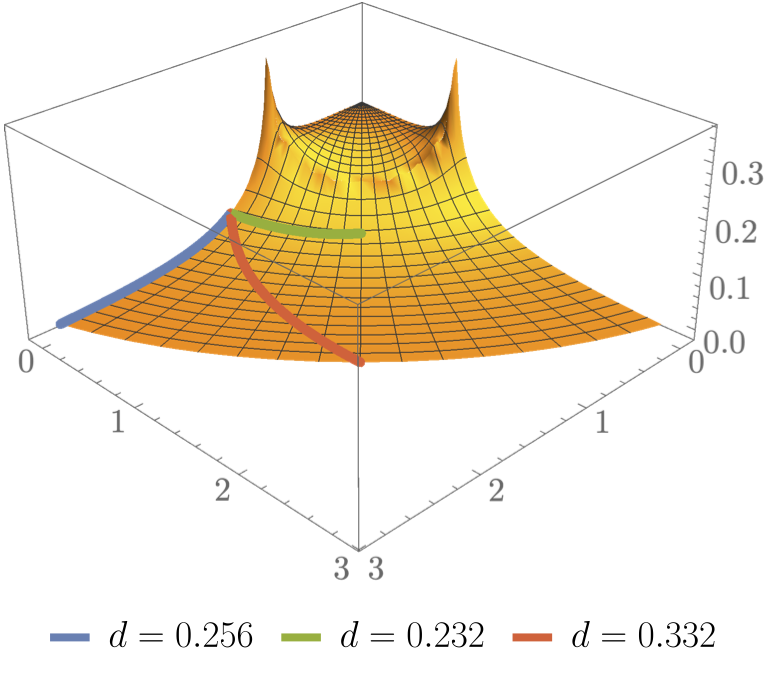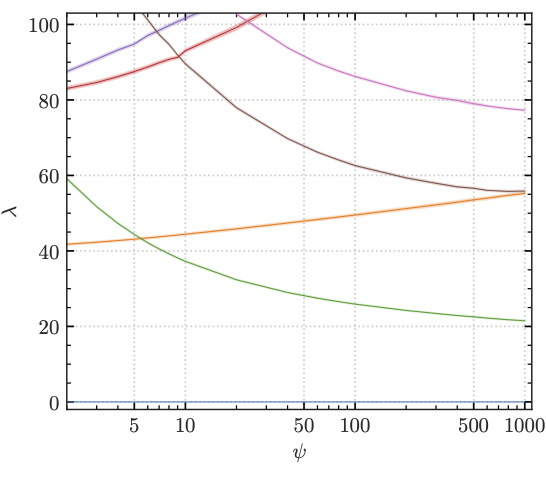Take for example the Klein-Gordon equation $$g^{ab}\partial_a\partial_b \varphi = m^2\varphi$$. We see that the massive spectrum depends on the metric. We can compute moduli-dependent Calabi-Yau metrics, and hence we can compute (moduli-dependent) massive KK states on Calabi-Yau manifolds. The swampland distance conjecture tells us that this KK tower should become exponentially light when moving $$O(1)$$ distance (in Planck units) in moduli space (see top image). In this paper, we compute geodesics in moduli space to see what it means to move $$O(1)$$ in terms of the parameters that describe the CY. For Kähler moduli, it means that the numerical value of the Kähler parameters $$t_i$$ changes indeed by $$O(1)$$. For complex structure moduli $$\psi$$, it means that the numerical value changes by $$O(1000)$$. This is clear from mirror symmetry, since $$t=\log\psi$$. We then compute the massive eigenmodes of the CY Laplacian as we vary the moduli and find that states become light as $$m_\text{KK} \sim e^{-0.45 d}$$, where $$d$$ is the distance in moduli space, so one can move 2-3 Planck units before the KK tower comes down one e-fold. I think this is the first and only way of determining this constant (for finite distance). This confirms the SDC on this manifold, but also shows that moving a few Planck units can be feasible. Finally, we observe a curious pattern in the eigenmodes: They exhibit level crossing, and eigenmodes that transform in larger irreps of the CY symmetry group become heavier, while those in small irreps become lighter (see bottom image). We have no understanding of this phenomenon. If you do, please tell us!

more...